# Chemistry formula for class 11 chapter- Equilibrium

## General characteristics of Equilibrium Involving Physical Processes:

• For liquid 3/4,33,3/44 vapor equilibrium, the vapor pressure is constant at a given temperature.
• For solid 3/4,33,3/44 liquid equilibrium, there is only one temperature, i.e., (melting point) at a melting point at which solid and liquid co-exist. If there is an isolated system, the mass of the two phases remains constant.
• The solubility of solids in liquid is constant at a particular temperature.
• For the dissolution of gases in liquids, the concentration of a gas in a liquid is proportional to the pressure (concentration) of the gas over the liquid.
• Equilibrium is possible only in a closed system at a given temperature.
• There is dynamic equilibrium but stable condition.
• All measurable properties of the system remain constant at equilibrium.
• The magnitude of such quantities at any stage indicates the extent to which the reaction has proceeded.

For the given general equation Kc & Kp

xA + yB⇄ mC + nD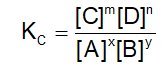where KC = equilibrium constant interms of concentration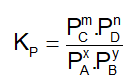Where KP = equilibrium constant interms of partial pressure

• Relation between KC & KP

Kp = Kc(RT)Δn

where Δn no. of moles of gaseous products-. No. of moles of gaseous reactants.

R = gas constant

T = Temperature in Kelvin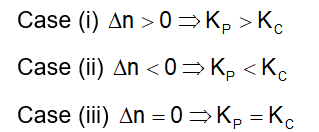• Equilibrium constant = KC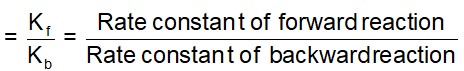• The expression for KC & KP for the equations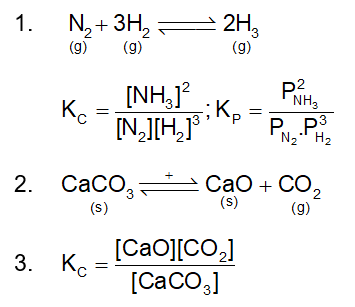But [CaCO3], [CaO] are taken as unity

Since CaCO3, CaO are solids

KC = [CO2]

### Equilibrim Formula

This approach comes from kinetics, as developed by Guldberg and Waage (1863). Equilibrium is known to have reached in a physical or chemical system when the rate of forwarding and reverse processes are equal.

At equilibrium,

Rate of forwarding reaction = Rate of backward reaction

### Law of Chemical Equilibrium

rf = rb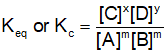Do follow NCERT textbook and solve questions with the help of Physics Wallah NCERT Solutions for class 11 chemistry. If any students need to take the online test to check their concepts or undertstanding then they can visit Quiz for Ionic and Chemical Equilibrium.

Download the free pdf sheet which consists of formulas and important points of Equilibrium from the link given below.